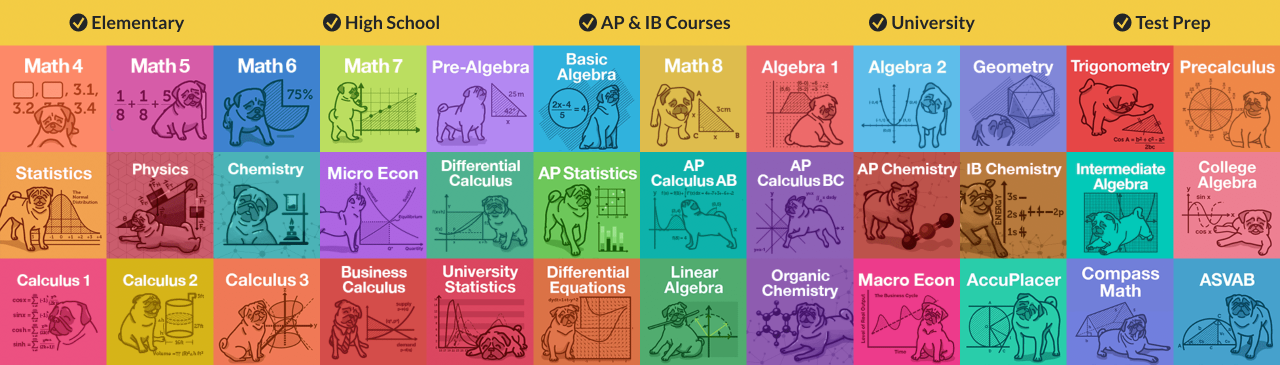# Calculus 2 Help & Practice

Our calculus tutors have you covered with our complete help for any calculus 2 courses that involve single-variable functions, whether it is Business Calculus 2, or IB Mathematics HL. Learn calculus 2 with ease!

Our comprehensive lessons on calculus 2 cover help on topics like Riemann Sum, Trig substitution, Alternating series test, Integral of trig functions, P series test, Integration by partial fractions and so much more. Learn the concepts with our online calculus 2 tutorials that show you step-by-step solutions to even the hardest integration calculus problems. Then, strengthen your understanding with tons of integration practice.

All our lessons are taught by experienced calculus 2 teachers. Let's finish your homework in no time, and ACE that final.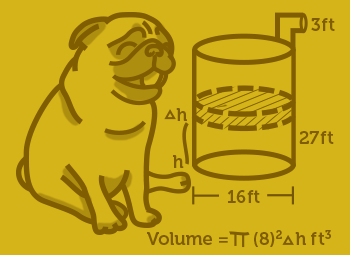#### Everything You Need in One Place

Homework problems? Exam preparation? Trying to grasp a concept or just brushing up the basics? Our extensive help & practice library have got you covered.#### Learn and Practice With Ease

Our proven video lessons ease you through problems quickly, and you get tonnes of friendly practice on questions that trip students up on tests and finals.#### Instant and Unlimited Help

Our personalized learning platform enables you to instantly find the exact walkthrough to your specific type of question. Activate unlimited help now!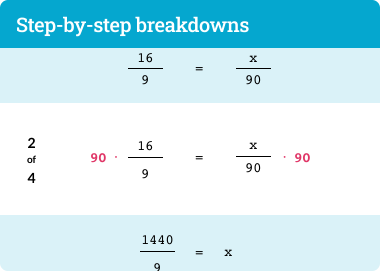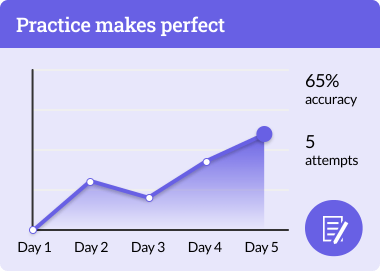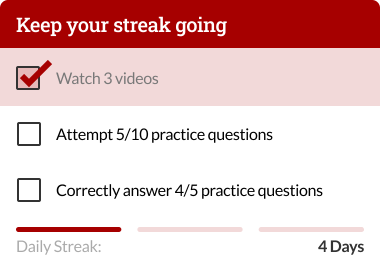## What is Calculus 2?

When it comes to learning Calculus 2, you'll need to come in with a solid understanding of the fundamentals of Calculus I as you won't go far in this second course without knowing the ins-and-outs of limits, derivatives and applications of derivative. Our Calculus 2 course outline is organized logically with comprehensive notes each step of the way.

For this course, our certified StudyPug Calculus 2 tutors will guide you through six topics:

• Integrals
• Integration Techniques
• Integration Applications
• Differential Equations
• Sequence and Series
• Parametric Equations and Polar Coordinates.

## Is Calculus 2 Hard?

Unless you are majoring in math, Calculus 2 will likely be the most difficult math course you will take thus far, mainly because it requires you to have a fair amount of maturity and creativity with math that you may not have needed up to this point. The hardship in understanding Calculus 2 isn't necessarily from committing the numerous formulas to memory, but instead, it can be difficult for knowing when to employ those Calculus 2 formulas. Sure, you're going to need to memorize all the fomulas, but you'll need to know the nuances of how the formulas operate, which in turn, helps you determine which Calculus 2 formula to use. It can be a dizzying amount of mental work that can be overwhelming for a student.

The perception of Calculus 2 being so difficult comes from the fact that a lot of students are actually surprisingly well prepared for Calculus 1 whereas this course often feels like a rude awakening. Compared to Calculus 1, it's relatively difficult to think of "real world application" that specifically depend on Calculus 2 concepts, and so there is a wide chasm between the material and students being able to relate to it. An easy Calculus 2 test can quickly turn into an impossible one when you start breaking down a nasty-looking integral that really only required a simple trig substitution.

Luckily, our Calculus 2 course is designed in a way where the complicated subject is broken into manageable chunks where we take time to walk you through those intricate nuances to bolster your grasp of the topic. If you're looking for Calculus 2 for dummies, we've got a smart solution that will turn you into a Calculus 2 master in no time.

## What comes after Calculus 2?

Firstly, it is highly recommended that you go through all of Calculus 1 before taking this Calculus 2. There's no real way around that as jumping into this course without a sound working knowledge of Calculus 1 is ill-advised. When anyone teaches Calculus 2, they will have to build on terms and formulas taught in Calculus 1. Besides that, another prerequisite for this course can be either Business Calculus or AP Calculus AB too.

After StudyPug has helped you conquer Calculus 2, your follow-up course should be either Calculus 3 or Linear Algebra where our instructors will further build on the knowledgebase you've gained from taking this course.

## Who needs to take Calculus 2?

In university, Calculus 2 is a required course for several academic disciplines including most science and math related majors like Physics, Engineering, Computer Science, etc. Basically, if you are in a field that uses math or science, you will absolutely encounter Calculus 2 in your course requirements.

Our Calculus 2 lessons are compatible with Calculus 2 textbooks such as Calculus: Early Transcendentals; Springer Calculus II, Pearson Calculus; and more.

## How to study for Calculus 2?

In StudyPug, our education philosophy revolves around explaining complicated topics like Calculus 2 in an approachable manner. Instead of trying to parse truths from Googling out specific topics you may have a missed a class on, you can come here at any time to learn from our Calculus 2 tutorials to catch up. When searching for Calculus 2 topics, be specific so we can be precise in helping out with that burning Calculus 2 question that's been on your mind.

## Here are just some of the reasons why you'll want to get Calculus 2 help with StudyPug:

• Our Calculus 2 tutorials are comprehensive, which means that we can answer any Calculus 2 questions that you may have. Whether it be Riemann Sum, Trig substitution, Alternating series test, Integral of trig functions, or P series test, StudyPug will provide answers you seek.
• Our Calculus 2 practice is available immediately after each Calculus 2 lesson for you to practise the lessons' material. After all, you can't master Calculus 2 without flexing that knowledge through practice.
• Our Calculus 2 tutorials are always available to help you for all your Calculus 2 needs. Even if you have to miss school from sickness or a vacation, you can always access these online Calculus 2 lessons which include an intro to the topic, practice, and a video tutorial with StudyPug 2 tutors.

## What do you learn in Calculus 2?

Calculus 2 is a bit of a mixed assortment of mathematical topics. The beginning of Calculus 2 deals with building on those aforementioned fundamentals of derivatives you should have touched on in Calculus 1.

You'll spend your early time formalizing antiderivatives, Riemann sums, the fundamental theorem, etc.

After that, you'll jump into integration techniques, which is usually the most difficult part of the course for most students. Here, you will pick up several different tricks to solve integration problems, but you'll also learn that integration is not as straightforward as differentiation.

From there, you'll then proceed to applications of integration to solve some very "fun" mathematical problems, like the area between two curves, volumes of different types of solids, average value of function, arc length, etc.

Next, you'll study differential equations where you will be exposed to an overview of the topic with its various solutions.

Following that, Sequence and Series is another heavy topic, but we divide it into 19 sub-topics so you can learn Calculus 2 at your own pace. Everything is covered here from an introduction to sequences to approximating functions with Taylor polynomials and error bounds.

Finally, we close out this course with a dive into Parametric Equations and Polar Coordinates. In this section, we teach you everything from how to define curves with parametric equations to understanding the arc length of polar curves.

If this sounds terrifying, fret not. StudyPug is committed to give you dedicated help with Calculus 2 questions by offering useful and easy-to-absorb Calculus 2 lessons.

## Using StudyPug for Calculus 2

Stuck on a whether to use u-substitution or numerical integration? Need to quickly look up which formula to use? Need an instant refresher on how to calculate the area between two curves? Well StudyPug can help you with any Calculus 2 problem as we offer thorough Calculus 2 homework help.

• To find the Calculus 2 topic where your Calculus 2 question belongs to, you can either use our search system to find the correct Calculus 2 topic, or just browse through the large list of Calculus 2 topics that we cover.
• Watch a step-by-step Calculus 2 tutorial within each topic to see how our Calculus 2 teacher solves the question along with his best Calculus 2 tips and tricks!
• If you are rusty on the Calculus 2 concept as a whole, it's always recommended to start by watching introduction to the topic for your Calculus 2 homework.
• Again, StudyPug serves up quality Calculus 2 homework help available for you around the clock.
• ###### I am taking a course called integration calculus in university. I am just wondering whether this calculus 2 course is useful for me.

Yes, you can find all the resources that you need for the integration calculus course here. We cover various topics on integral and more. If you are having integration problem or looking for integral practices, you are in the right place for help.

• ###### I am studying for the calculus 2 final. How can StudyPug help me prepare for it?

Everyone has their own way of studying. However, practice makes perfect is always true. Therefore, you should take advantage of the calculus 2 problems available on our site. You can work on those problems and see if you need help on a specific topic. Once you identify the topic, watch our video lessons and then do the problems again.

• ###### What are the prerequisites for Calculus 2?

A prerequisite for this course is either Calculus 1 or AP Calculus AB, and after you mastered Calculus 2, your follow up course should be either Calculus 3 or Linear Algebra.

• ##### Students and parents love our math help
###### But don't take our word for it…
•Carson E.
parent

When we saw our son's grades we looked online for a convenient, affordable and effective solution. StudyPug has been a great investment.

•Jason G.
high school senior

This website saved my butt last semester. I am using it againthis semester. Dennis is the best online tutor... I also like that I can watch videos over and over until I really understand the concept. If you want to save time, sign up...it's only ten bucks and it saved me hours of study time. Thanks, Dennis!

•Aaron M.
high school student

I get a whole library of videos that covers everything from basic to complex mathematics for a very low monthly price. Excellent videos, easy-to-understand and most of all they work. My math test results are 10 points higher than last semester.

• See all our Testimonials